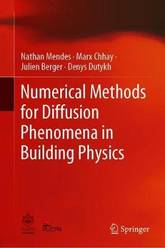#### Nathan Mendes author

Image credit: dr. shordzi, on Flickr
Filter
(found 1 product)This book is the second edition of Numerical methods for diffusion phenomena in building physics: a practical introduction originally published by PUCPRESS (2016). It intends to stimulate research in simulation of diffusion problems in building physics, by providing an overview of mathematical models and numerical techniques such as the finite ...
Numerical Methods for Diffusion Phenomena in Building Physics: A Practical Introduction
This book is the second edition of Numerical methods for diffusion phenomena in building physics: a practical introduction originally published by PUCPRESS (2016). It intends to stimulate research in simulation of diffusion problems in building physics, by providing an overview of mathematical models and numerical techniques such as the finite difference and finite-element methods traditionally used in building simulation tools. Nonconventional methods such as reduced order models, boundary integral approaches and spectral methods are presented, which might be considered in the next generation of building-energy-simulation tools. In this reviewed edition, an innovative way to simulate energy and hydrothermal performance are presented, bringing some light on innovative approaches in the field.